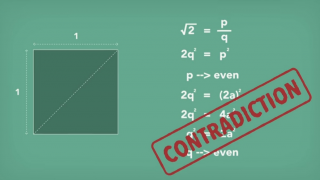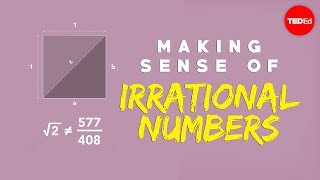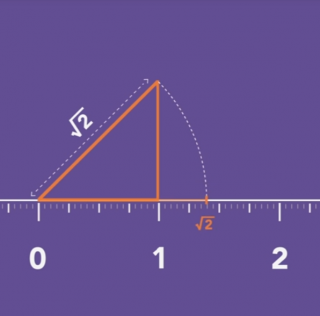BC 0500? Hippasus
According to Pythagoras theorem the diagonal length of a square with each side measuring one unit would be square root of two. The assumption that square root of two could be expressed as a ratio of two integers deduces a contradiction.
http://qindex.info/i.php?x=502601 Hippasus
○ According to Pythagoras theorem the diagonal length of a square with each side measuring one unit would be square root of two. ○ The assumption that square root of two could be expressed as a ratio of two integers deduces a contradiction.
http://qindex.info/i.php?x=6563√2 ≈ 1.414
According to Pythagoras theorem the diagonal length of a square with each side measuring one unit would be square root of two. The assumption that square root of two could be expressed as a ratio of two integers deduces a contradiction. #math
http://qindex.info/i.php?x=9592
-----